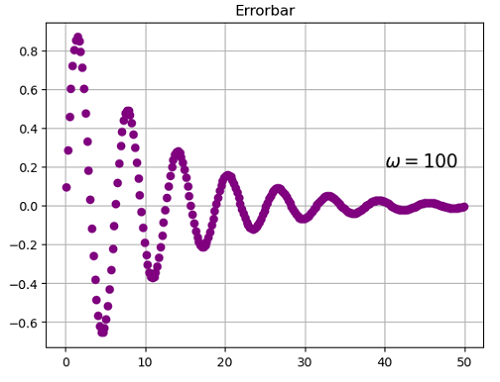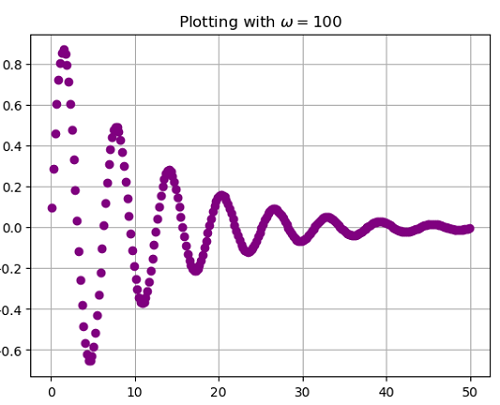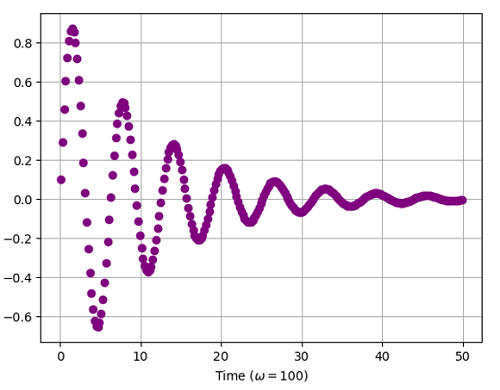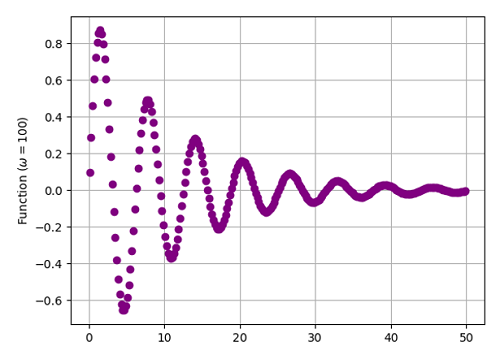# Python | Adding Omega in Plot Label

In this article, we are going to learn how to add 𝜔 (Omega) letter in the plot label in Python?
Submitted by Anuj Singh, on July 18, 2020

𝜔 is very oftenly used greek mathematical letters and has a higher repetition in probability. In this article, we are going to add 𝜔 using a command in matplotlib.

plt.text(3, 0.4, r'$\omega=100$')plt.title('Errorbar with 'r'$\omega=100$')
#Adding 𝜔 in title of the figureplt.xlabel('Time ('r'$\omega=100)$')
#Adding 𝜔 in title of the figureplt.ylabel('Variation ('r'$\omega=100)$')
#Adding 𝜔 in title of the figure## Python code for adding omega in plot label

import numpy as np
import matplotlib.pyplot as plt

x = np.arange(0.1, 50, 0.2)
y = np.sin(x)*np.exp(-0.09*x)

# In text
plt.figure()
plt.plot(x,y, 'o', color='purple')
plt.title('Errorbar')
plt.text(40, 0.2, r'$\omega=100$', fontsize=15)
plt.grid()
plt.show()

# In title
plt.figure()
plt.plot(x,y, 'o', color='purple')
plt.title('Plotting with 'r'$\omega=100$')
plt.grid()
plt.show()

# In x-axis label
plt.figure()
plt.plot(x,y, 'o', color='purple')
plt.xlabel('Time ('r'$\omega=100)$')
plt.grid()
plt.show()

# In y-axis label
plt.figure()
plt.plot(x,y, 'o', color='purple')
plt.ylabel('Function ('r'$\omega=100)$')
plt.grid()
plt.show()


Output:

Output is as figure